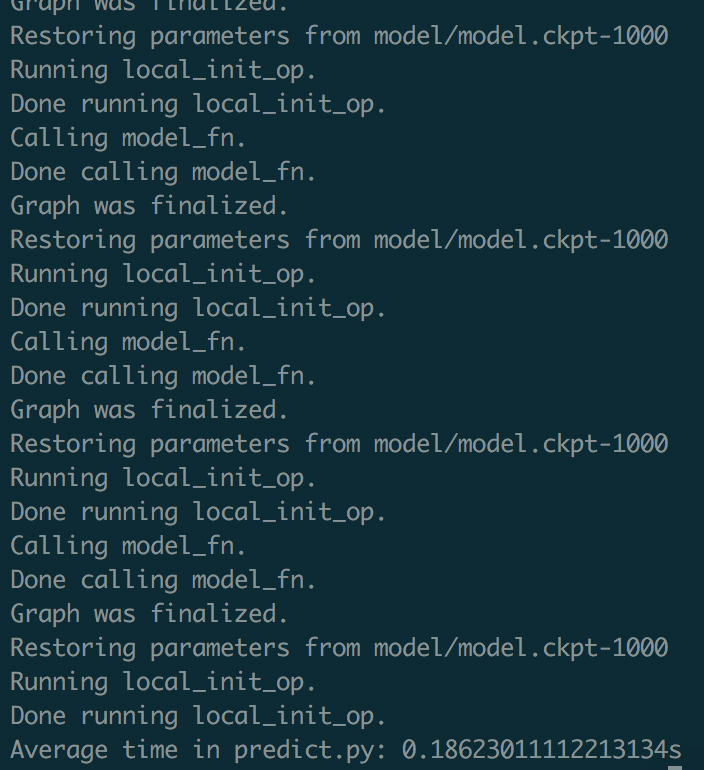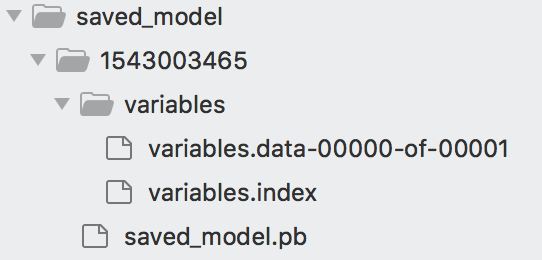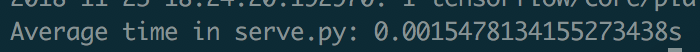Code available on github. A more advanced use of the techniques covered in this article can also be found here.

Topics tf.estimator, serving_input_receiver_fn, ServingInputReceiver, export_saved_model, contrib.predictor, from_saved_model

## Introduction

The tf.estimator framework is really handy to train and evaluate a model on a given dataset. In this post, I show how a simple tensorflow script can get a state-of-the-art model up and running.

However, when it comes to using your trained Estimator to get predictions on the fly, things get a little bit messier.

This blog post demonstrates how to properly serialize, reload a tf.estimator and predict on new data, by going over a dummy example (fully reproductible by cloning the github repo) and get a 100x speedup over the vanilla implementation.

Good news is: in the end, it is dead simple and only takes a few lines of code 😎.

## Model

Let’s say that we have trained an estimator that computes

We model this as a simple dense layer with one output. In other words, our model has 2 parameters a and b to learn such that

Using the tf.estimator paradigm, here is our model_fn

def model_fn(features, labels, mode, params):
if isinstance(features, dict):  # For serving
features = features['feature']

predictions = tf.layers.dense(features, 1)

if mode == tf.estimator.ModeKeys.PREDICT:
return tf.estimator.EstimatorSpec(mode, predictions=predictions)
else:
loss = tf.nn.l2_loss(predictions - labels)
if mode == tf.estimator.ModeKeys.EVAL:
return tf.estimator.EstimatorSpec(
mode, loss=loss)

elif mode == tf.estimator.ModeKeys.TRAIN:
loss, global_step=tf.train.get_global_step())
return tf.estimator.EstimatorSpec(
mode, loss=loss, train_op=train_op)
else:
raise NotImplementedError()


If you think this is a rather preposterous use of Tensorflow and Deep Learning in general, why not have a look at this very serious article on a related topic?

If you need an introduction to tf.estimator, you can read my introduction with an emphasis on NLP here.

## Training the Estimator

To train our model, we generate fake data using tf.data (here is a short yet comprehensive introduction to tf.data).

def train_generator_fn():
for number in range(100):
yield [number, number], [2 * number]

def train_input_fn():
shapes, types = (2, 1), (tf.float32, tf.float32)
dataset = tf.data.Dataset.from_generator(
train_generator_fn, output_types=types, output_shapes=shapes)
dataset = dataset.batch(20).repeat(200)
return dataset


Once we have our model_fn and our train_input_fn, training our tf.estimator is a matter of 2 lines of code.

estimator = tf.estimator.Estimator(model_fn, 'model', params={})
estimator.train(train_input_fn)


As expected, training takes a few seconds and manages to learn the parameters a and b as indicated by a very small loss.

At the end of the training, because we specified the model_dir argument of the Estimator, the model directory contains full checkpoints of the graph.

## Reload and Predict (first attempt)

Now, let’s say that we have a service, exterior to our model, that keeps sending us new data. Everytime we receive a new example, we want to run our model. Let’s fake the service by using a python generator

def my_service():
for number in range(100, 110):
yield number


Because we don’t know in advance our full dataset, our code needs to look like

for number in my_service():
prediction = get_prediction(number)


Imagine a Flask app that calls the get_prediction function everytime some new data is sent to some url.

Let’s use the predict method of the tf.estimator.Estimator class.

We first create a special input_fn that formats the new number for the Estimator

def example_input_fn(number):
dataset = tf.data.Dataset.from_generator(
lambda: ([number, number] for _ in range(1)),
output_types=tf.float32, output_shapes=(2,))
iterator = dataset.batch(1).make_one_shot_iterator()
next_element = iterator.get_next()
return next_element, None


This is the same input_fn as the one used for training except that this time the data generator only yields the number sent by our service.

And we can get predictions by doing

for nb in my_service():
example_inpf = functools.partial(example_input_fn, nb)
for pred in estimator.predict(example_inpf):
print(pred)


The predict method returns a generator. Because our dataset only yields one example, the loop is executed only once and it seems like we achieved our goal: we used the estimator to predict the outcome on new data.

## The problem

Now, let’s have a look at the logs.What? Everytime we call predict, our estimator instance reloads the weights from disk! Thus, it takes an astonishing 0.19s per loop execution! There must be a better way… Keep in mind that this model only has 2 parameters, what will happen when you have a BFN*?

*starts with Big, ends with Network

## A clever fix?

If you have good python skills, you might notice that everything in this pipeline seems to be built on top of python generators. We could control the iteration of these pipelined generators using the next method.

This clever solution has been investigated by some people, see here for instance.

The idea, overall, is to build the predict generator on top of the service generator (like Russian dolls) and move to the next prediction when we receive a new example. However, apart from being hacky, this method has a downside: chaining 2 generators is not as reliable, as the iteration of the second might depend on a batch of the first. In other words, the estimator.predict seems to be built with some kind of batching mechanism, which, in the end, causes problems (and I don’t want to have to look at the details of this method, as I shouldn’t have to).

Even if there are some workarounds and you could probably make this work eventually, it requires custom hacks depending on your data pipeline.

Now, let’s explore a much better option (which also seems to be the official one, even though the guides and documentation are pretty scarce and vague about the subject. But this sadly won’t come as a surprise and is also the existential motivation of this very blog post).

## Exporting the estimator as a tf.saved_model

Tensorflow provides a more efficient way of serializing any inference graph that plays nicely with the rest of the ecosystem, like Tensorflow Serving.

In line with the tf.estimator technical specifications of making it an easy-to-use, high-level API, exporting an Estimator as a saved_model is really simple.

We first need to define a special input_fn (as always we can’t expect the estimator to guess how to format the data).

def serving_input_receiver_fn():
"""Serving input_fn that builds features from placeholders

Returns
-------
"""
number = tf.placeholder(dtype=tf.float32, shape=[None, 1], name='number')
receiver_tensors = {'number': number}
features = tf.tile(number, multiples=[1, 2])


The user will provide number to the model, which will be fed to the placeholder and transformed to features. Ultimately, the model will receive a dictionary {'feature': features}. NB: the data that the user provides in that case is already batched.

Then, reloading and serializing the estimator is straightforward

estimator = tf.estimator.Estimator(model_fn, 'model', params={})


For each new export, you will find a new time-stamped subdirectory (here 1543003465), containing the graph definition as a protobuffer (saved_model.pb) along with the weights (in variables/). If your graph uses other resources (like vocab files for lookup tables, that sort of thing), you will also find them under an asset/ directory.## Reload and Predict (the good way)

While the saved model can be used directly by tools like Tensorflow serving, some people (including me) might want to reload it in python. After all, maybe your application is built in Flask.

First, let’s find the latest set of weights by exploring the subdirectories under saved_model

export_dir = 'saved_model'
subdirs = [x for x in Path(export_dir).iterdir()
if x.is_dir() and 'temp' not in str(x)]
latest = str(sorted(subdirs)[-1])


If you don’t know / don’t use the pathlib module (python3 only), try using it. It bundles a lot of os.path functionnality (and more) in a much nicer and easy-to-use package. I started using it after reading about it on this blog which also has a lot of other excellent articles.

Once we have found the directory containing the latest set of weights, we can use a predictor to reload the weights. I heard from this very simple (yet incredibly powerful) class on this stackoverlow answer.

from tensorflow.contrib import predictor

predict_fn = predictor.from_saved_model(latest)
for nb in my_service():
pred = predict_fn({'number': [[nb]]})['output']


The predictor class also comes with a from_estimator method!

Under the hood, this predictor class implementation uses all the tools mentionned in the official guide. It uses a tf.saved_model.loader to load the tf.saved_model into a session, reloads the serving signature from the protobuffer, extract the input and output tensors of the graph and bundles everything in a nice callable for ease of use.

We could probably have gleaned enough information here and there in the official documentation and hack it ourselves, but a better implementation is already there. Why not advertise it more on the official guide? Anyway, a big thank you to the developers that made it available!

## Conclusion and next steps

It is still a mystery to me why the tf.estimator API does not offer an efficient predict method for on-the-fly requests, or at least advertise a bit more the tools covered in this blog post. After all, with a few lines of code you get an even better result! The relative lack of documentation and official guides is not new: it is probably really hard to keep up with the rapid evolution of the framework while still offering comprehensive and coherent documentation to its users.

Using the predictor as explained above yields a 100x speedup on our dummy example!If you’re curious to see a more “real-life” use of these methods, my other repo using tf.estimator for NER (Named Entity Recognition using a bi-LSTM + CRF) implements them all!